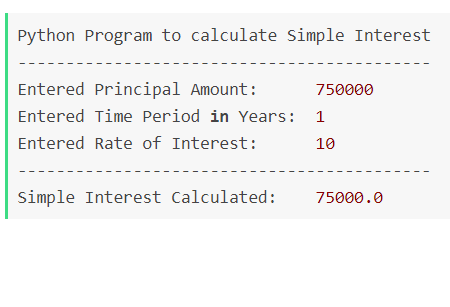# Python Program To Calculate Simple Interest (SimpleInterest.py)

Before getting into the code of calculating Simple Interest in Python, lets understand how simple interest is calculated.

## What is Simple Interest?

Simple Interest is the amount of interest charge that you need to pay to the bank when you borrow money from them (a loan). There are three important variables that you would need to understand when writing your python program.

1. Principal Amount (P): Principal is the amount that you have borrowed from the bank or the base price of the good or service that you have purchased and applied for a loan. Example if you brought a mortgage for \$75,0000 USD than this amount is your principal.
2. Interest Rate (R): Interest rate is the price you need to pay to the bank for the Principal (Loan Amount) you borrowed. This is usually calculate annually. Interest is calculate in percentage. Example for a mortgage loan of \$750,000 USD if the internet rate is 10% for a year (annual interest) then you need to pay \$75,000 interest every year.
3. No of days (N): This is the tenor of the loan amount that you and the counter party have agreed upon.

## Formula for Simple Interest

Simple Interest = (P [Principal Amount] X N [No of days] X R [Interest Rate]) / 100

## Python code for Simple Interest calculation: SimpleInterest.py

``````# This is a Python program to calculate
# Simple Interest  for the inputted
# Principal Amount, Rate of Interest,
# and Time frame
# Formula: (P x N x R)/100
#
# author: Code2care.org

def simple_interest_function(principal_amount,time_period_in_years,rate_of_interest):

print("Python Program to calculate Simple Interest")
print("-------------------------------------------")

print("Entered Principal Amount:     ", principal_amount)
print("Entered Time Period in Years: ", time_period_in_years)
print("Entered Rate of Interest:     ",rate_of_interest)

#Formula for Simple Interest
simple_interest =  (principal_amount * time_period_in_years * rate_of_interest)/100

print('-------------------------------------------')
print("Simple Interest Calculated:   ", simple_interest)

simple_interest_function(750000, 1, 10) ``````
Output:
``````Python Program to calculate Simple Interest
-------------------------------------------
Entered Principal Amount:      750000
Entered Time Period in Years:  1
Entered Rate of Interest:      10
-------------------------------------------
Simple Interest Calculated:    75000.0``````